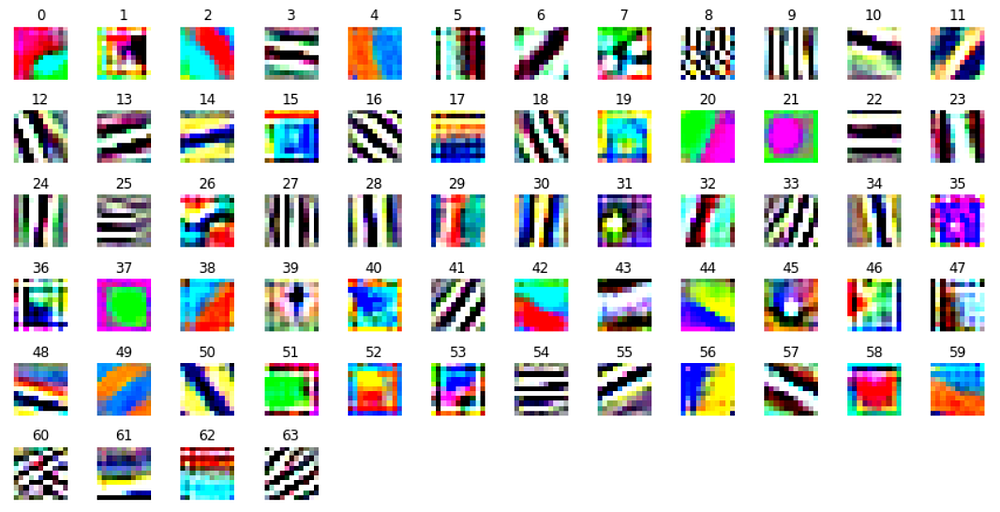# Visualizing Convolution Neural Networks using Pytorch

Source: Deep Learning on MediumConvolution Neural Network (CNN) is another type of neural network that can be used to enable machines to visualize things and perform tasks such as image classification, image recognition, object detection, instance segmentation etc…But the neural network models are often termed as ‘black box’ models because it is quite difficult to understand how the model is learning the complex dependencies present in the input. Also, it is difficult to analyze why a given prediction is made during inference.

In this article, we will look at two different types of visualization techniques such as :

1. Visualizing learned filter weights.
2. Performing occlusion experiments on the image.

These methods help us to understand what does filter learn? what kind of images cause certain neurons to fire? and how good are the hidden representations of the input image?.

# Receptive Field of Neuron

Before we go ahead and visualize the working of Convolution Neural Network, we will discuss the receptive field of filters present in the CNN’s.

Consider that we have a two-layered Convolution Neural Network and we are using 3×3 filters through the network. The centered pixel marked in the yellow present in Layer 2 is actually the result of applying convolution operation on the center pixel present in Layer 1 (by using 3×3 kernels and stride = 1). Similarly, the center pixel present in Layer 3 is a result of applying convolution operation on the center pixel present in Layer 2.

From the above figure, we can see that each filter channel out of a total of 64 filters (0–63) is visualized separately. For eg. figure 0,0 indicate that the image represents the zeroth filter corresponding to the zeroth channel. Similarly, figure 0,1 indicates that the image represents the zeroth filter corresponding to the first channel and so on.

Visualizing the filter channels individually gives more intuition about what different filters are trying to learn based on the input data. By looking closely at the filter visualizations, it is clear that the patterns found in some of the channels from the same filter are different. That means not all channels present in a filter are trying to learn the same information from the input image. As we move deeper into the network the filter patterns more complex, they tend to capture high-level information like the face of a dog or cat.

As we go deeper and deeper into the network number of filters used for convolution increases. It is not possible for us to visualize all these filter channels individually either as a single image or each channel separately because of the large number of such filters. The second convolution layer of Alexnet (indexed as layer 3 in Pytorch sequential model structure) has 192 filters, so we would get 192*64 = 12,288 individual filter channel plots for visualization. Another way to plot these filters is to concatenate all these images into a single heatmap with a greyscale.

`#plotting single channel imagesplot_weights(alexnet, 0, single_channel = True, collated = True)`
`#plotting single channel images - second convolution layerplot_weights(alexnet, 3, single_channel = True, collated = True)`
`#plotting single channel images - third convolution layerplot_weights(alexnet, 6, single_channel = True, collated = True)`

As you can see there are some interpretable features like edges, angles, and boundaries in the images from the first convolution layer. But as we go deeper into the network it becomes harder to interpret the filters.